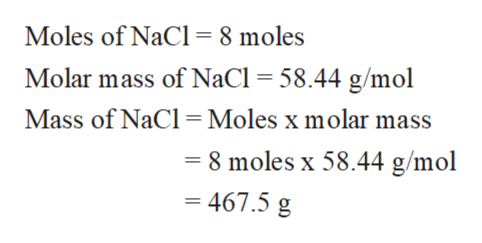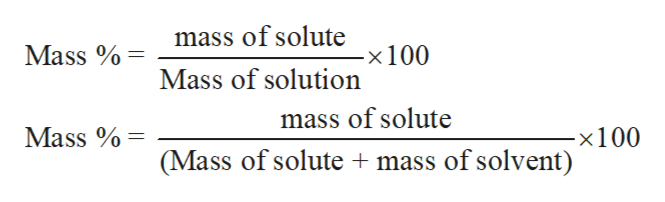# A 8.0 m NaCl solution would have what mass percent of NaC in the solution? The molar mass of NaCl is 58.44g.

Question
5 views

A 8.0 m NaCl solution would have what mass percent of NaC in the solution? The molar mass of NaCl is 58.44g.

check_circle

Step 1

Molality is the way to express the concentration of any solution. It can be defined as the number of moles of solute dissolves in 1 kg of the solvent. The mathematical expression for molality can be written as:

Step 2

8 m solution or 8 molal solution of NaCl means 8 moles of NaCl dissolve in 1 kg of solvent. Calculate the mass of NaCl from the given moles and molar mass:help_outlineImage TranscriptioncloseMoles of NaCl 8 moles Molar mass of NaCl = 58.44 g/mol Mass of NaCl Moles x molar mass -8 moles x 58.44 g/mol = 467.5 g fullscreen
Step 3

Mass percent or weight percent of a solution is the mass of the solute by the mas...help_outlineImage Transcriptionclosemass of solute Mass -x 100 Mass of solution mass of solute -x 100 mass of solvent) Mass (Mass of solute fullscreen

### Want to see the full answer?

See Solution

#### Want to see this answer and more?

Solutions are written by subject experts who are available 24/7. Questions are typically answered within 1 hour.*

See Solution
*Response times may vary by subject and question.
Tagged in
ScienceChemistry

### Solutions